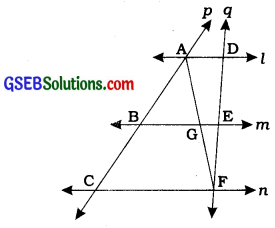# GSEB Class 9 Maths Notes Chapter 8 Linear Equations in Two Variables

This GSEB Class 9 Maths Notes Chapter 8 Linear Equations in Two Variables covers all the important topics and concepts as mentioned in the chapter.

## Linear Equations in Two Variables Class 9 GSEB Notes

If no three points out of four coplanar points are collinear, then a closed figure formed by joining these four points in order is called a quadrilateral.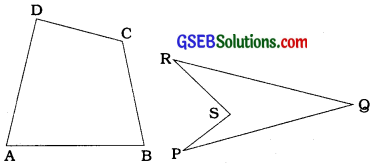By joining four points in order, we get two types of quadrilaterals as shown in the above figures. Quadrilateral ABCD is called a convex quadrilateral while quadrilateral PQRS is called a concave quadrilateral.
We are going to study only convex quadrilateral. Hence, whenever we use the word quadrilateral, it stands for convex quadrilateral.

A quadrilateral has four sides, four angles and four vertices.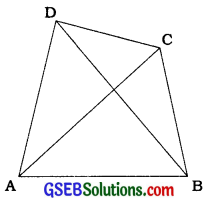In quadrilateral ABCD, AB, BC, CD and DA are four sides, ∠A, ∠B, ∠C and ∠D are four angles and A, B, C and D are four vertices.
By joining two opposite vertices of a quadrilateral, we get a diagonal of the quadrilateral. A quadrilateral has two diagonals. In quadrilateral ABCD, AC and BD are diagonals.

Four sides, four angles and two diagonals are called the parts (elements) of a quadrilateral. Thus, a quadrilateral has 10 parts.

Angle sum property of a quadrilateral:
The sum of angles of a quadrilateral is 360°.
So, in quadrilateral XYZW,
∠X + ∠Y + ∠Z + ∠W = 360°.

• Trapezium: If the sides in only one pair * of opposite sides of a quadrilateral are parallel, then the quadrilateral is called a trapezium.
• Parallelogram: If the sides in both the pairs of opposite sides of a quadrilateral are parallel, then the quadrilateral is called a parallelogram.
• Rectangle: If one angle (hence all angles) of a parallelogram is a right angle, then it is called a rectangle.
• Rhombus: If all the sides of a parallelogram are equal, then it is called a rhombus.
• Square: A parallelogram, in which one angle (hence all angles) is a right angle and all the sides are equal, is called a square.
• Kite: A quadrilateral in which sides of two pairs of adjacent sides are equal but no opposite sides are equal or parallel Is called a kite.

Properties of a parallelogram:

• Theorem 8.1: A diagonal of a parallelogram divides it into two congruent triangles.
• Theorem 8.2: In a parallelogram, opposite sides are equal.
• Theorem 8.3: If each pair of opposite sides of a quadrilateral is equal, then it is a parallelogram.
• Theorem 8.4: In a parallelogram, opposite angles are equal.
• Theorem 8.5: If in a quadrilateral, each pair of opposite angles is equal, then it is a parallelogram.
• Theorem 8.6:The diagonals of a parallelogram bisect each other.
• Theorem 8.7: If the diagonals of a quadrilateral bisect each other, then it is a parallelogram.
• Theorem 8.8: A quadrilateral is a parallelogram if a pair of opposite sides is equal and parallel.

In a quadrilateral if (1) both the pairs of opposite sides are equal or (2) both the pairs of opposite angles are equal or (3) the diagonals bisect each other or (4) the sides in one pair of opposite sides are equal and parallel, then that quadrilateral is a parallelogram.

Rectangle:
The diagonals of a rectangle are equal and bisect each other. The converse of the above statement is also true.

Rhombus:
The diagonals of a rhombus bisect each other at right angles.
The converse of the above statement is also true.

Square:
The diagonals of a square are equal and bisect each other at right angles.
The converse of the above statement is also true.Kite:
In a kite, one diagonal is a part of the perpendicular bisector of the other diagonal.
The converse of the above statement is also true.

Example 1:
Show that each angle of a rectangle is a right angle.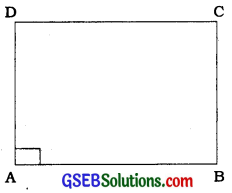Let, ABCD be a parallelogram in which ∠A = 90°. S
Here, AD ∥ BC and AB is a transversal. <
∴ ∠A + ∠B = 180° J
(Interior angles on the same side of the ? transversal) S
But, ∠A = 90°
∴ ∠B = 180° – ∠A = 180° – 90° = 90°
Now, ∠C = ∠A and ∠D = ∠B
(Opposite angles of the parallelogram)
∴∠C = 90° and ∠D = 90°.

Therefore, each of the angles of ABCD is a s right angle.
Since ABCD is a parallelogram with ∠A = 90°, by definition it is a rectangle.

Hence, each angle of a rectangle is a right angle.

Example 2:
Show that the diagonals of a rhombus are perpendicular to each other.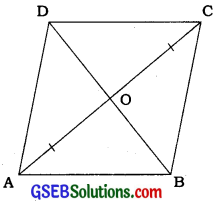Consider the rhombus ABCD (see the given figure).
You know that AB = BC = CD = DA (All the sides of a rhombus are equal.)
Now, in ∆ AOD and ∆COD,
OA = OC (Diagonals of a parallelogram bisect each other)
OD = OD (Common)
∴ ∆ AOD ≅ ∆COD (SSS congruence rule)
∴ ∠AOD = ∠ COD (CPCT)
But, ∠ AOD + ∠COD = 180° (Linear pair)
∴ 2∠AOD = 180°
∴ AOD = 90°
So, the diagonals of a rhombus are perpendicular to each other.

Example 3:
ABC is an isosceles triangle in which AB = AC. AD bisects exterior angle PAC and CD II AB (see the given figure).
Show that (i) ∠DAC = ∠ BCA and
(ii) ABCD is a parallelogram.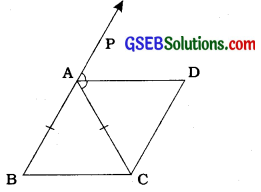(i) ∆ ABC is isosceles in which AB = AC
(Given)
∴∠ABC = ∠ACB (Angles opposite to equal sides)
Also, ∠ PAC = ∠ ABC + ∠ ACB (Exterior angle of a triangle)
∴ ∠ PAC = 2 ∠ ACB (1)
Now, AD bisects ∠PAC.
∴ ∠PAC = 2 ∠DAC (2)
Then, from (1) and (2), we get 2 ∠DAC = 2 ∠ACB
∴∠DAC = ∠BCA

(ii) Now, these equal angles form a pair of alternate angles when line segments BC and AD are intersected by transversal AC.
∴ BC ∥ AD
Also, BA ∥ CD (Given)
Now, both pairs of opposite sides of quadrilateral ABCD are parallel.
So, ABCD is a parallelogram.

Example 4:
Two parallel lines l and m are intersected by a transversal p (see the given figure). Show that the quadrilateral formed by the bisectors of interior angles is a rectangle.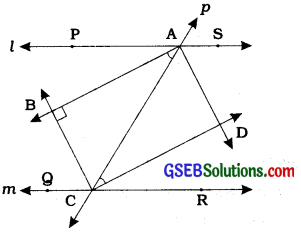It is given that PS ∥ QR and transversal p intersects them at points A and C respectively. The bisectors of ∠PAC and ∠ACQ intersect at B and the bisectors of ∠ACR and ∠SAC intersect at D.
Now, ∠PAC = ∠ACR (Alternate angles as l ∥ m and p is a transversal)
∴ $$\frac{1}{2}$$ ∠PAC = $$\frac{1}{2}$$∠Z ACR
∴ ∠ BAC = ∠ACD
These form a pair of alternate angles for lines AB and DC with AC as transversal and they sire equal.
∴ AB ∥ DC
Similarly, BC ∥ AD (Considering ∠ACB and ∠CAD)
Therefore, quadrilateral ABCD is a parallelogram.
Also, ∠PAC + ∠CAS = 180° (Linear pair)
∴ $$\frac{1}{2}$$ ∠ PAC + $$\frac{1}{2}$$∠CAS = $$\frac{1}{2}$$ × 180° = 90°
∴ ∠BAC + ∠CAD = 90°
∴ ∠BAD = 90°
Thus, ABCD is a parallelogram in which one angle is 90°. Therefore, ABCD is a rectangle.

Example 5.
Show that the bisectors of angles of a parallelogram form a rectangle.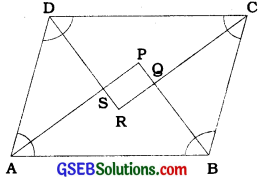Let R Q, R and S be the points of intersection of the bisectors of ∠A and ∠B, ∠B and ∠C, ∠C and ∠D, and ∠D and ∠A respectively of parallelogram ABCD (see the given figure). Since DS bisects ∠D and AS bisects ∠A,
∠DAS + ∠ADS = $$\frac{1}{2}$$∠A + $$\frac{1}{2}$$∠D
= $$\frac{1}{2}$$(∠A + ∠D)
= $$\frac{1}{2}$$ × 180° (∠A and ∠D are interior angles on the same side of the transversal)
= 90°
Also, ∠DAS + ∠ADS + ∠DSA = 180°
(Angle sum property of a triangle) 90° + ∠DSA = 180°
∠DSA = 90°
∴ ∠PSR = 90° (Vertically opposite angles)

Similarly, it can be shown that ∠APB = 90° or ∠SPQ = 90°
Similarly, ∠PQR = 90° and ∠SRQ = 90°
So, PQRS is a quadrilateral in which all angles are right angles.
We have shown that ∠PSR = ∠PQR = 90° and ∠SPQ = ∠SRQ = 90°. So both pairs of opposite angles are equal.

Therefore, PQRS is a parallelogram in which one angle (in fact all angles) is 90° and hence, PQRS is a rectangle.Example 6:
ABCD is a parallelogram in which P and Q are midpoints of opposite sides AB and CD (see the given figure). If AQ intersects DP at S and BQ intersects CP at R, show that:
(i) APCQ is a parallelagrair.
(ii) DPBQ is a parallelogram.
(iii) PSQR is a parallelogram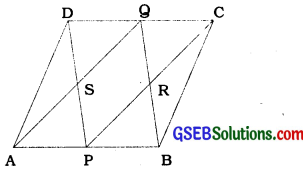(i) In quadrilateral APCQ,
AP ∥ QC (v AB ∥ CD) ……..(1)
AP = $$\frac{1}{2}$$AB,
CQ = $$\frac{1}{2}$$CD (Given)

Also, AB = CD (Opposite sides of a parallelogram)
So, AP = QC ……………(2)
Therefore, APCQ is a parallelogram.
[From (1) and (2) and theorem 8.8]
∴AQ ∥CP

(ii) Similarly, quadrilateral DPBQ is a parallelogram, because
DQ ∥ PB and DQ = PB
∴ DP ∥ QB

(iii) In quadrilateral PSQR,
SP ∥ QR (∵ DP ∥ QB)
Similarly, SQ ∥ PR (∵ AQ ∥ CP)
So, PSQR is a parallelogram.

Properties of diagonals of quadrilaterals:
Following properties are proved in the sums of Exercise:

• The diagonals of a parallelogram bisect each other.
• The diagonals of a rhombus bisect each other at right angles.
• The diagonals of a rectangle are equal and bisect each other.
• The diagonals of a square are equal and bisect each other at right angles.

The converse of each of the four statements given above are also true.

The midpoint theorem:
Theorem 8.9:
The line segment joining the midpoints of two sides of a triangle is parallel to the third side and is half of the third side.

Theorem 8.10:
The line drawn through the midpoint of one side of a triangle, parallel to another side bisects the third side.

• The quadrilateral obtained by joining in order the midpoints of the sides of any convex quadrilateral is a parallelogram.
• The quadrilateral obtained by joining in order the midpoints of the sides of a parallelogram is a parallelogram.
• The quadrilateral obtained by joining in order the midpoints of the sides of a rhombus is a rectangle.
• The quadrilateral obtained by joining in order the midpoints of the sides of a rectangle is a rhombus.
• The quadrilateral obtained by joining in order the midpoints of the sides of a square is a square.

Example 1:
In A ABC, D, E and F are respectively the midpoints of sides AB, BC and CA (see the given figure). Show that ∆ABC is divided into four congruent triangles by joining D, E and F.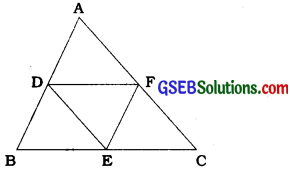As D and E are midpoints of sides AB and BC of the triangle ABC, by theorem 8.9,
DE ∥ AC
Similarly, DF ∥ BC and EF ∥ AB
Therefore, ADEF; BDFE and DFCE are all parallelograms.

Now, DE is a diagonal of parallelogram BDFE,
∆ BDE ≅ ∆FED

Similarly, ∆ DAF = ∆ FED and
∆ EFC ≅ ∆ FED So, all the four triangles are congruent.Example 2:
l, m and n are three parallel lines intersected by transversals p and q such that I, m and n cut off equal intercepts AB and BC on p (see the given figure). Show that Z, m and n cut off equal intercepts DE and EF on q also.